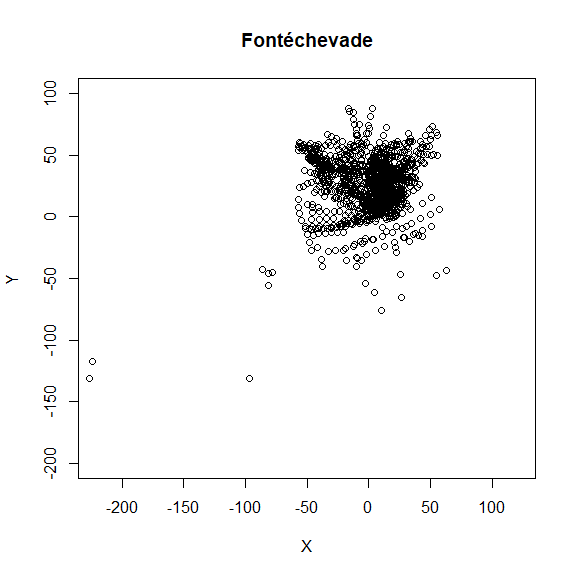The following code demonstrates how R can be used to read the Fontéchevade SQLite database for plotting and analysis.

knitr::opts_chunk$set(echo = TRUE, warning = FALSE) library(plyr) library(dplyr) library(RSQLite) library(knitr) Read the tables from the database into dataframes. Then merge the context and xyz tables together, and merge the lithics and context tables (so that Layer becomes part of the lithics table). Note that an example is not given here for the smallfinds, but the table is opened anyway here for example. con = DBI::dbConnect(RSQLite::SQLite(), dbname = "data/fc.sqlite") context = dbReadTable(con, "fc.context") %>% select(-unit, -idno) xyz = dbReadTable(con, 'fc.xyz') %>% mutate(squid = squid_id) lithics = dbReadTable(con, "fc.lithics") %>% mutate(squid = squid_id) smallfinds = dbReadTable(con, "fc.small_finds") dbDisconnect(con) fc = join(context, xyz, by = c('squid'), type = 'right') lithics = join(lithics, context, by = c('squid'), type = 'left') Make a plot of the location of the items in the database using only Suffix = 0 points. plot(fc$x[fc$suffix == 0], fc$y[fc$suffix == 0], asp = 1, main = 'Fontéchevade', xlab = 'X', ylab = 'Y', xlim = c(-200, 100), ylim = c(-200, 100))Provide a breakdown of the basic types of lithics in the database. kable(table(Dataclass = lithics$dataclass))
Dataclass Freq
COMPFLAKE 2084
COMPTOOL 119
CORE 249
COREFRAG 37
CORETOOL 9
DISTFLAKE 134
DISTTOOL 10
MEDFLAKE 57
MEDTOOL 47
PROXFLAKE 876
PROXTOOL 26